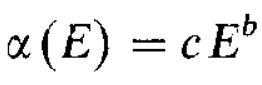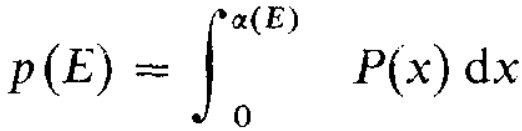# Calling Integral when defining a TF1

Hello rooters,

I’m trying to define the following functions:

1.2.where `P(x)` is a chi-square distribution with one degree of freedom.

What I’ve done so far is the following:

``````TF1 *f_Porter_Thomas = new TF1("f_Porter_Thomas", "ROOT::Math::chisquared_pdf(x,1,0)",0,10);
TF1 *f_alpha = new TF1("f_alpha", "*pow(x,)" , E_low, E_high);

``````

and it’s nothing complicated. I’m having hard time, though, defining a `TF1` the would include `TF1::Integral()`.

So for example this doesn’t work:

`TF1 *f_p = new TF1("f_p" , "f_Porter_Thomas->Integral(0, 10)", E_low, E_high);`

What I get is:

``````input_line_321:2:105: error: member reference type 'double' is not a pointer
Double_t TFormula____id9601315710509570316(Double_t *x){ return (ROOT::Math::chisquared_pdf(x,1,0))->Integral(0,10) ; }
~~~~~~~~~~~~~~~~~~~~~~~~~~~~~~~~~~~~~~  ^
input_line_322:2:105: error: member reference type 'double' is not a pointer
Double_t TFormula____id9601315710509570316(Double_t *x){ return (ROOT::Math::chisquared_pdf(x,1,0))->Integral(0,10) ; }
~~~~~~~~~~~~~~~~~~~~~~~~~~~~~~~~~~~~~~  ^
Error in <prepareMethod>: Can't compile function TFormula____id9601315710509570316 prototype with arguments Double_t*
``````

Eventually I would like to be able to define the `TF1` like so:

`TF1 *f_p = new TF1("f_p" , "f_Porter_Thomas->Integral(0, <f_alpha(x)>)", E_low, E_high);`

Any idea on how to do that?

`TF1 *f_p = new TF1("f_p", [=](double *x, double *p){ return f_Porter_Thomas->Integral(0., f_alpha->Eval(x)); }, E_low, E_high, 0);`